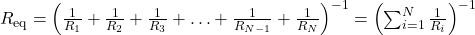Have you encountered a situation where during New Year’s Eve, the lighting in your house goes off just because of one faulty LED? Well, you are not alone!

This is a classic example which would persuade us to read series vs parallel circuits.

Current: Current is flow of charge (electrons)

Circuit: A closed/complete path which would enable the electrons to flow across is a circuit.

Resistance: Just like Air offers resistance in the motion of a particle in air (recall physics 101), likewise any equipment which resists the flow of current is called Electrical resistance. It can be offered by elements such as Resistors, Capacitors, Inductors etc.

Resistance is measured with SI unit Ohm while Capacitance is measured with SI unit Farad.

Voltage: The potential is termed as the voltage. More commonly used term, voltage difference is the difference across any two points.

Let’s talk about Series and Parallel circuit:

Series connection: As the same suggests, when the connections (usually of Resistors, Capacitors and Inductors) are one after the other is called series connection. Here’s the behavior with respect to different types of elements:

For resistors: Current in all the resistors remain the same while voltage drop across each depends on its value.

Total value of resistance is found by adding the individual resistances:For capacitors: Charge in all the capacitors remain the same while the potential drop across each depends on the capacitance.

The reciprocal of equivalent capacitance is equal to the sum of reciprocal of individual capacitance.Parallel connection: As the same suggests, when the connections (usually of Resistors, Capacitors and Inductors) are such that one end point of all the elements is connected to other-another while the other end point is also connected to one-another by a continuous wire of negligible resistance. Here’s the behavior with respect to different types of elements:

For resistors: Voltage across all the resistors remain the same while current across each depends on its value.

The reciprocal of equivalent resistance is equal to the sum of reciprocal of individual resistance.For capacitors: Voltage across all the capacitors remain the same while the charge on each depends on the capacitance.

Total value of capacitance is found by adding the individual capacitances:Interesting enough? We noticed that series and parallel connection of Resistors and Capacitors is a mixed bag wherein there are some similarities along with some differences.

I have covered various questions in this video.